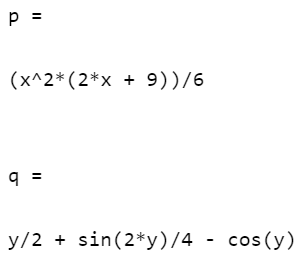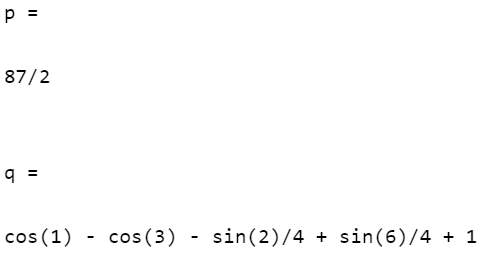Open In App

# Integration in MATLAB

Integration is defined as the process of finding the anti derivative of a function. It is used to calculate area, volume, displacement, and many more. In this article, we will see how to perform integration on expressions in MATLAB.

There are two types of Integration:

• Indefinite integral: Let f(x) be a function. Then the family of all antiderivatives is called the indefinite integral of a function f(x) and it is denoted by ∫f(x)dx. The symbol ∫f(x)dx is read as the indefinite integral of f(x) with respect to x. Thus ∫f(x)dx= ∅(x) + C. Thus, the process of finding the indefinite integral of a function is called the integration of the function.
• Definite integrals: Definite integrals are the extension after indefinite integrals, definite integrals have limits [a, b]. It gives the area of a curve bounded between given limits. It is denoted by ∫f(x)dx under the limit of a and b, it denotes the area of curve F(x) bounded between a and b, where a is the lower limit and b is the upper limit.

Before moving to Integration, we first need to assign an expression to a variable in MATLAB which can be done by using the inline() function. It creates a function to contain the expression.

Syntax:

f = inline(expr, var)

Here f is the inline function created to contain the expression, expr can be any expression and var is the variable in that expression.

Now, after assigning the expression using the inline() function, we need to integrate the expression. This task can be performed using the int() function. The int() function is used to integrate expressions in MATLAB.

Syntax:

int(f,v)

Parameters:

• f: Expression
• v: Variable

## Indefinite Integral

Indefinite integrals are those integrals that do not have any limit and containing an arbitrary constant.

Step-wise Approach:

Step 1: Use Inline function for the creation of the function for integration.

## Matlab

 `% create a inline function``f=inline(``'x^2+3*x'` `,``'x'``);``g=inline( ``'sin(y) + cos(y)^2'``, ``'y'``);`

Step 2: Create a symbolic function.

## Matlab

 `% create a symbolic function``syms x;``syms y;`

Step 3: Use int to find out the integration.

## Matlab

 `% for the integration use int() ``% and pass the parameters``% (expression,  variable)``% variable= variable for integration``p=int (f(x),x);``q=int (g(y),y);`

Complete Code:

## Matlab

 `% create a inline function``f=inline(``'x^2+3*x'` `,``'x'``);``g=inline( ``'sin(y) + cos(y)^2'``, ``'y'``);`` ` `% create a symbolic function``syms x;``syms y;`` ` `% variable= variable for``% integration``p=int (f(x),x);``q=int (g(y),y);`` ` `% display``p``q`

Output## Definite Integral

Definite integrals are those integrals that have an upper limit and a lower limit. Let’s take the above example and add the limits.

Step-wise Approach:

Step 1: Use the Inline function for the creation of the function for integration.

## Matlab

 `% create a inline function``f=inline(``'x^2+3*x'` `,``'x'``);``g=inline( ``'sin(y) + cos(y)^2'``, ``'y'``);`

Step 2: Create a symbolic function.

## Matlab

 `% create a symbolic function``syms x;``syms y;`

Step 3: Use int to find out the integration and pass down the values of the lower limit, upper limit.

## Matlab

 `%for the integration use int() ``% and pass the parameters``%(expression, lower limit, ``% upper limit)``p=int (f(x),1,4);``q=int (g(y),1,3);`` ` `% use double to know the value``% in decimal``double(q);`

Complete Code:

## Matlab

 `% create a inline function``f=inline(``'x^2+3*x'` `,``'x'``);``g=inline( ``'sin(y) + cos(y)^2'``, ``'y'``);`` ` `% create a symbolic function``syms x;``syms y;`` ` `% for the integration use int() and pass the parameters``% (expression, lower limit, upper limit)``p=int (f(x),1,4);``q=int (g(y),1,3);``double(q);`` ` `% display``p``q`

Output: